# Which of the following equations are dimensionally consistent?

Which of the following equations are dimensionally consistent?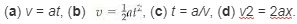(A) Consider the equation v = at
Carry out a dimension analysis on both sides.
The dimension of the left-hand side =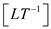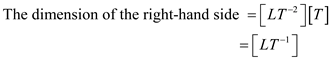The dimensions on both sides are the same.
Thus, the equation v = at is dimensionally consistent.

(B) Consider the equation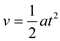Write the dimensions on both sides.
The dimension of the left-hand side =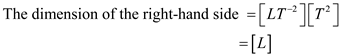The dimensions on both sides are different.
Thus, the equation is dimensionally inconsistent.

( C) Consider the equation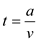Carry out a dimension analysis on both sides.
The dimension of the left-hand side = [ T ]The dimensions on both sides are different.
Thus, the equation is dimensionally inconsistent.

(D) Consider the equation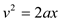Carry out a dimension analysis on both sides.
The dimension of the left-hand side =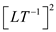=The dimension of the right-hand side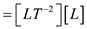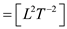The dimensions on both sides are the same.
Thus, the equation is dimensionally consistent.

masteringphysics